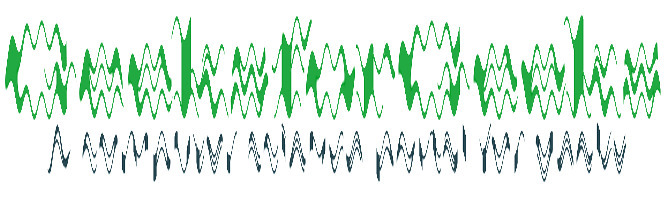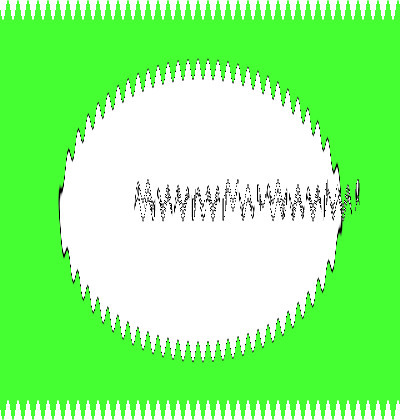# Wand wave() function – Python

The wave() function is an inbuilt function in the Python Wand ImageMagick library which is used to alter an image along with a sine wave. It creates a ripple effect.

Syntax:

`wave(amplitude, wave_length, method)`

Parameters: This function accepts three parameters as mentioned above and defined below:

• Amplitude: This parameter stores the value of the amplitude of the sine wave.
• Wave_length: This parameter stores the value of the wavelength of the sine wave.
• method: This parameter is used to specify the value of method which takes pixel interpolation method as ‘undefined’, ‘average’, ‘average9’, ‘average16’, ‘background’, ‘bilinear’, ‘blend’, ‘catrom’, ‘integer’, ‘mesh’, ‘nearest’, ‘spline’

Return Value: This function returns the Wand ImageMagick object.

Original Image:Example 1:

 `# Import library from Image  ` `from` `wand.image ``import` `Image ` ` `  `# Import the image ` `with Image(filename ``=``'../geeksforgeeks.png'``) as image: ` `    ``# Clone the image in order to process ` `    ``with image.clone() as wave: ` `        ``# Invoke wave function with amplitude as 12, wavelength as 15 ` `        ``# method as 'bilinear' ` `        ``wave.wave(``12``, ``15``, ``'bilinear'``) ` `        ``# Save the image ` `        ``wave.save(filename ``=``'wave1.jpg'``) `

Output:Example 2:

 `# Import libraries from the wand   ` `from` `wand.image ``import` `Image ` `from` `wand.drawing ``import` `Drawing ` `from` `wand.color ``import` `Color ` ` `  `with Drawing() as draw: ` `    ``# Set Stroke color the circle to black ` `    ``draw.stroke_color ``=` `Color(``'black'``) ` `    ``# Set Width of the circlw to 2  ` `    ``draw.stroke_width ``=` `1` `    ``# Set the fill color to 'White (# FFFFFF)' ` `    ``draw.fill_color ``=` `Color(``'white'``) ` ` `  `    ``# Invoke Circle function with center at 50, 50 and radius 25 ` `    ``draw.circle((``200``, ``200``), ``# Center point ` `                ``(``100``, ``100``)) ``# Perimeter point ` `    ``# Set the font style ` `    ``draw.font ``=` `'../Helvetica.ttf'` `    ``# Set the font size  ` `    ``draw.font_size ``=` `30` `     `  `    ``with Image(width ``=` `400``, height ``=` `400``, background ``=` `Color(``'# 45ff33'``)) as pic: ` `        ``# Set the text and its location ` `        ``draw.text(``int``(pic.width ``/` `3``), ``int``(pic.height ``/` `2``), ``'GeeksForGeeks !'``) ` `        ``# Draw the picture ` `        ``draw(pic) ` `        ``# Invoke wave() function with amplitude as 10, wavelength as 10 ` `        ``# method as 'blend' ` `        ``pic.wave(``10``, ``10``, ``'blend'``) ` `        ``# Save the image  ` `        ``pic.save(filename ``=``'wave2.jpg'``) `

Output:My Personal Notes arrow_drop_upCheck out this Author's contributed articles.

If you like GeeksforGeeks and would like to contribute, you can also write an article using contribute.geeksforgeeks.org or mail your article to contribute@geeksforgeeks.org. See your article appearing on the GeeksforGeeks main page and help other Geeks.

Please Improve this article if you find anything incorrect by clicking on the "Improve Article" button below.

Article Tags :

Be the First to upvote.

Please write to us at contribute@geeksforgeeks.org to report any issue with the above content.